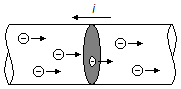Solved Problem on Electric CurrentPortuguêsEnglish

A current of 2 A flows in a wire, knowing that the elementary charge is 1.6 × 10−19. How many electrons passing through a cross-section of the wire during a time interval of 1 s?

Problema data
• electric current:    i = 2 A;
• elementary charge:    e = 1.6 × 10−19 C;
• time interval:    t = 1 s.
Problem diagram

In figure 1 the electrons move from left to right across a cross-section, highlighted in gray, the conventional current ( i ) is indicated in the direction opposite to the movement of the electrons.figure 1

Solution

The electric current is the rate at which charge flows through the cross-section
$\bbox[#99CCFF,10px] {i=\frac{\Delta q}{\Delta t}} \tag{I}$
The amount of charge that crosses a given cross-section, is the product of the number of charges, that crosses the section, by the elementary charge
$\bbox[#99CCFF,10px] {\Delta q=ne} \tag{II}$
substituting the expression (II) in (I), we have
$i=\frac{ne}{\Delta t}\\ n=\frac{i\;\Delta t}{e}$
substituting the data of the problem
$n=\frac{2 \times 1}{1.6 \times 10^{-19}}\\ n=\frac{2 \times 10^{19}}{1.6}$
$\bbox[#FFCCCC,10px] {n=1.25 \times 10^{19}\;\rm{el{\acute e}trons}}$